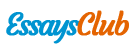# Chem Lab - Density

Autor:   •  March 22, 2018  •  568 Words (3 Pages)  •  138 Views

Page 1 of 3

...

Mean Density: .7272 Absolute Deviation .15245

Average value density = .7272+ or - .152456 g/mL at 20 degrees celsius

Percent Precision = 20%

Range = .9384-.5811 g/mL

The percent composition of my unknown is 20% Ethanol, 80% water.

Summary: Unknown solution was found to be .7272 +or- .15245 g/mL at 20 degrees celsius

Percent precision is 20% and the range is .9384 to .5811 g/mL

Calculations:

Part A:

Part B:

Discussion:

In this procedure I needed to obtain the densities of irregular shaped solids and liquids. For part A of the experiment, I needed to find the mass of the unknown metal and also find the volume of the metal. I then drew my graph and found the slope of the line which gave me the density of the unknown substance. The evidence from my data and calculations suggest that my unknown substance was mossy tin. My ending results were accurate to the known values.

In part B of the experiment I needed to find the percentage of ethanol and water in a unknown solution. In order to do that I had to find the density of the solution by finding the mass of the solution and the volume of the solution. Then using the formula d/v, and also calculating the mean density, absolute deviation which therefore lead me to find the composition of the unknown solution which ended up being 20% ethanol and 80% water. I additionally graphed a calibration curve to further get a better understanding of my results.

Error Analysis:

In this experiment there was room for error, especially in calculations and precise measurements. It is nearly impossible to gather exact numbers because there are other contributing factors such as air bubbles in the cylinder, which could in the end affect the density.

One other possible source of error could have been the temperature of solution/substance because colder temperature has a greater density than a warmer solution. In all, my measurements and calculations could have been affected by these slight errors.

...